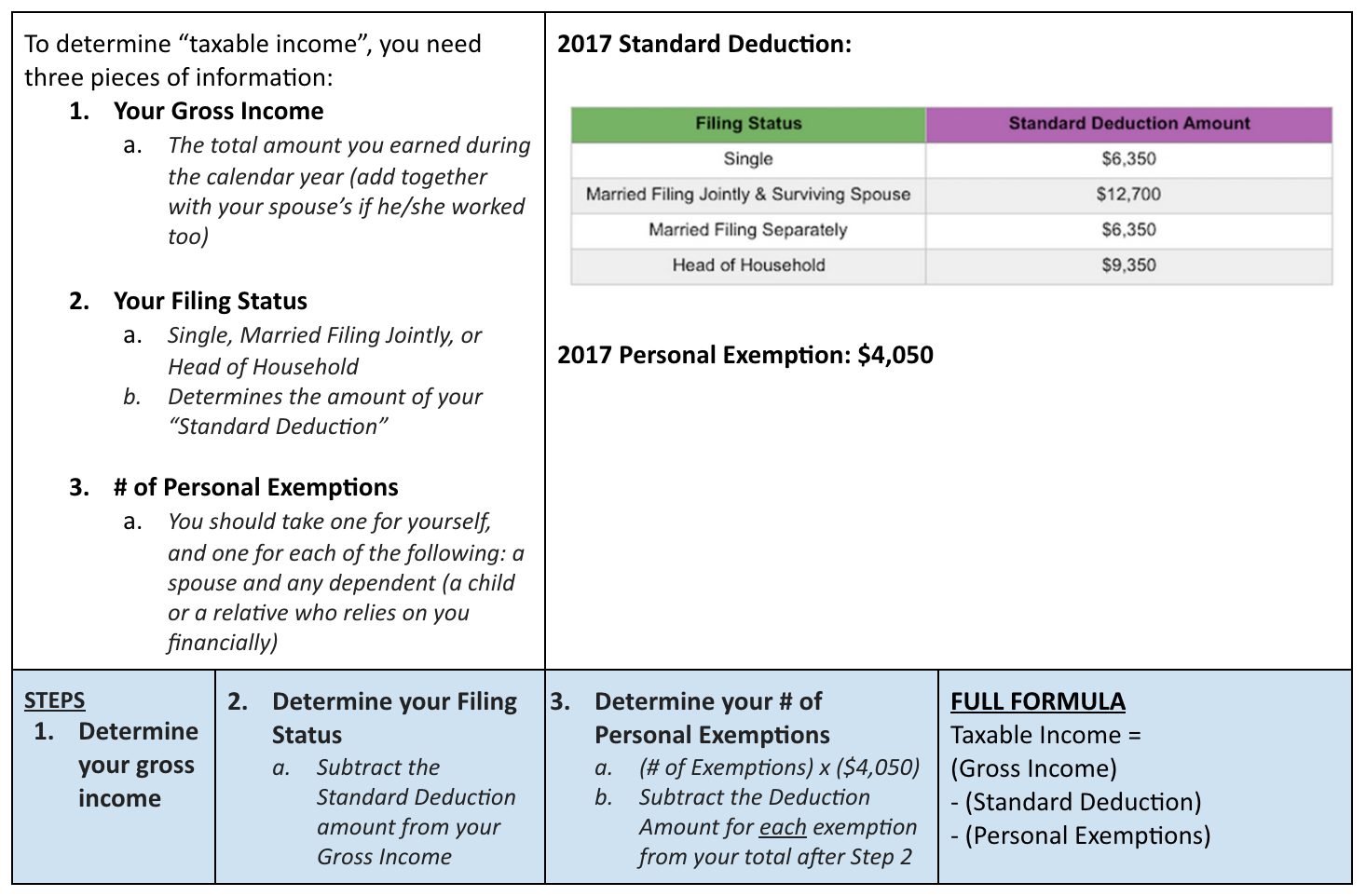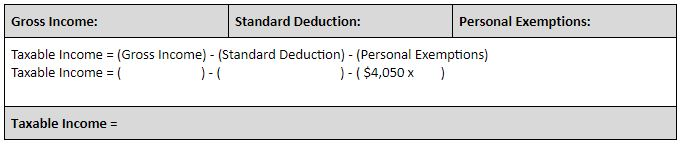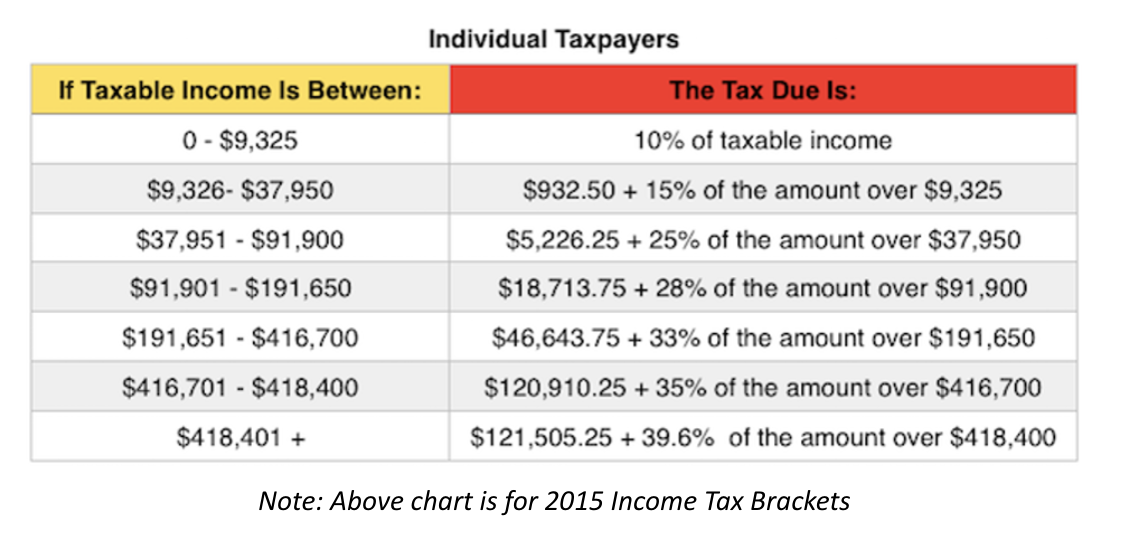starstarstarstarstarstarstarstarstarstar
by Megan Heine
| 6 Questions
In this activity, you will go in-depth to see how a tax bill is calculated. There are two main components we will cover
Part I: Calculating your Taxable Income
One nice thing about the U.S. tax system is you aren’t taxed on your entire income. You are allowed to use “deductions” and “exemptions” to reduce your “taxable income,” which reduces the amount you pay in taxes.Calculate the taxable income for a single person with a gross income of \$50,3001
1
What is the Taxable Income for a single person with a gross income of \$50,300?
Calculate the taxable income for a single person with a gross income of \$75,0002
1
What is the taxable income for a single person with a gross income of \$75,000?
Calculate the taxable income for a married couple with a combined gross income of \$100,0003
1
What is the taxable income for a married couple with a combined gross income of \$100,000?
Part II: Calculating your Tax Due
In the United States, we have a “progressive tax system,” meaning the more you make, the higher taxes you pay.
Use the chart below to answer the questions:What is the total tax due for someone with a taxable income of \$100,000? What is their marginal tax rate? Effective tax rate?4
1
Calculate the tax due and the effective tax rate for a single filer whose taxable income is \$100,000.
What is the total tax due for a single filer with a gross income of 100,000? What is their marginal tax rate? Effective tax rate? (REMEMBER you have to calculate the taxable income first)
5
1
What is the taxable income for a single filer with a gross income of \$100,000?6
1
Calculate the tax due and the effective tax rate for a single filer whose gross income is \$100,000. (You will use the taxable income from the question above)